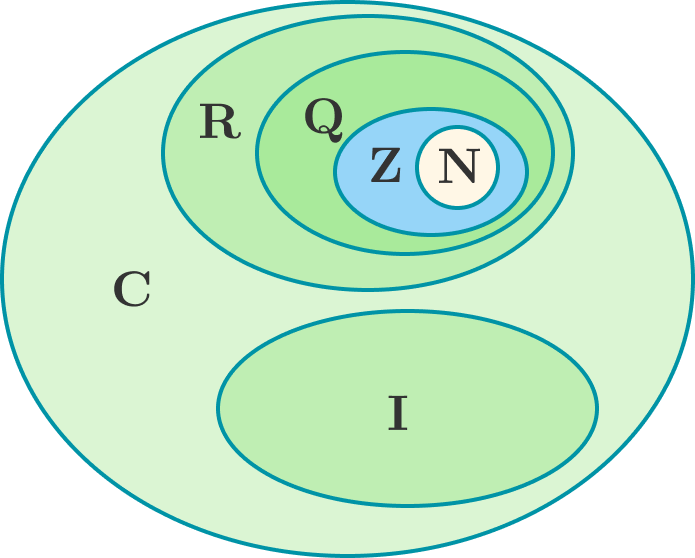# Find the truth - Humanity at stake1) $\frac { e }{ \pi }$ is a rational number.
2) $\frac { \pi }{ e }$ is an irrational number.
3) $\frac { \pi+e }{ e }$ is a rational number.

Give your answer as the mean of the serial numbers of the statements which are true.
$\big($E.g., if all statements are true, the answer is $\frac { 1+2+3 }{ 3 } =2.\big)$


Details and Assumptions:

• $e$ may not necessarily be the exponential constant and $\pi$ may not necessarily be equal to 3.14159...
×